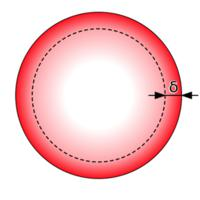Reviews of Modern Physics ( IF 38.296 ) Pub Date : 2019-06-25 , DOI: 10.1103/revmodphys.91.021003
Gabriele La Nave, Kridsanaphong Limtragool, and Philip W. PhillipsA theory of fractional electricity and magnetism is presented here which is capable of describing phenomena as disparate as the nonlocality of the Pippard kernel in superconductivity and anomalous dimensions for conserved currents in holographic dilatonic models. While it is a standard result in field theory that the scaling dimension of conserved currents and their associated gauge fields are determined strictly by dimensional analysis and hence cannot change under any amount of renormalization, it is also the case that the standard conservation laws for currents, dJ=0, remain unchanged in form if any differential operator that commutes with the total exterior derivative, [d,Y^]=0, multiplies the current. Such an operator, effectively changing the dimension of the current, increases the allowable gauge transformations in electromagnetism and is at the heart of Nöther’s second theorem. However, this observation has not been exploited to generate new electromagnetisms. Here a consistent theory of electromagnetism is developed that exploits this hidden redundancy in which the standard gauge symmetry in electromagnetism is modified by the rotationally invariant operator, the fractional Laplacian. The resultant theories are shown to all allow for anomalous (nontraditional) scaling dimensions of the gauge field and the associated current. Using the Caffarelli-Silvestre theorem [Caffarelli, L., and L. Silvestre, 2007, Commun. Partial Differ. Equations 32, 1245.], its extension [La Nave, G., and P. Phillips, 2017, arXiv:1708.00863 [Commun. Math. Phys. (in press)] ] to p forms and the membrane paradigm, either the boundary (UV) or horizon (IR) theory of holographic dilatonic models are shown to both be described by such fractional electromagnetic theories. The nonlocal Pippard kernel introduced to solve the problem of the Meissner effect in elemental superconductors can also be formulated as a special case of fractional electromagnetism. Because the holographic dilatonic models produce boundary theories that are equivalent to those arising from a bulk theory with a massive gauge field along the radial direction, the common thread linking both of these problems is the breaking of U(1) symmetry down to Z2. The standard charge quantization rules fail when the gauge field acquires an anomalous dimension. The breakdown of charge quantization is discussed extensively in terms of the experimentally measurable modified Aharonov-Bohm effect in the strange metal phase of the cuprate superconductors.

down
wechat
bug